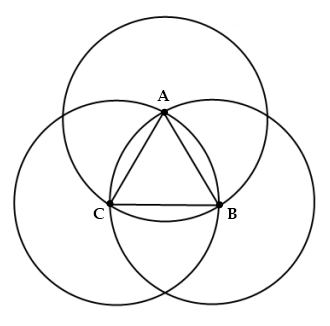# Three Circles

Geometry Level 3Circles $A$, $B$, and $C$ are shown above. The difference between the lengths of major arc $CB$ and minor arc $CB$ is $\dfrac {8π} {3}$. What is the area of $\triangle ABC$?

×